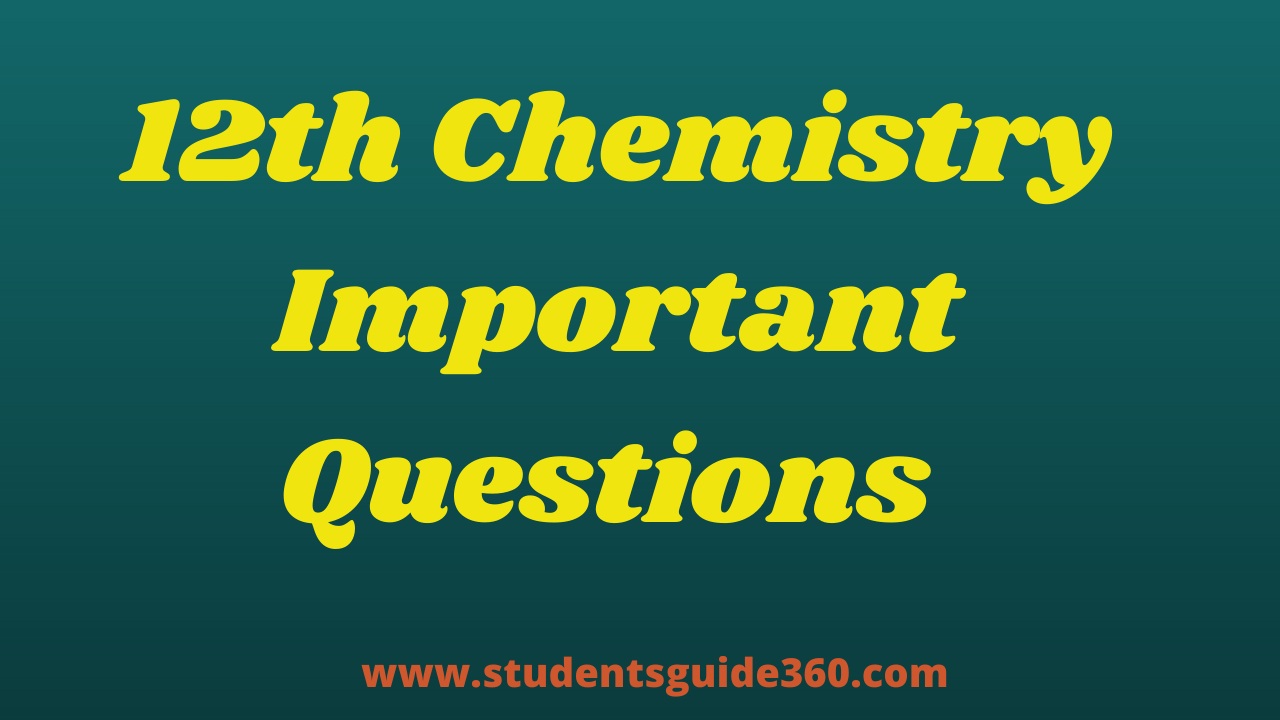# 12th Chemistry Important Questions Unit 7 Chemical Kinetics

TN 12th Chemistry Important Questions Unit 7 Chemical Kinetics. 12th Chemistry Important Questions Chapter 7 Transition And Inner Transition Elements +2 Chemistry Important 2 Marks, 12th Chemistry Important 3 Marks, Chemistry Important 5 Marks Questions Based on reduced Syllabus 2021-2022. 12th Free Online Test (MCQs). HSC 12th Chemistry Revision Test Important Questions. 12th Tamil Full Guide.## Unit 7 Chemical Kinetics

### 12th Chemistry All Units Important Questions

• 1. Define average rate and instantaneous rate.
• 2. Define rate law and rate constant.
• 3. Derive integrated rate law for a zero-order reaction A –> product.
• 4. Define the half-life of a reaction. Show that for a first-order reaction half-life is independent of initial concentration.
• 5. What is an elementary reaction? Give the differences between the order and molecularity of a reaction.
• 6. Write the rate law for the following reactions.
(a) A reaction that is 3/2 order in x and zero-order in y.
(b) A reaction that is second order in NO and first order in Br2.
• 7. The rate law for a reaction of A, B, and C has been found to be
rate = K[A]2[B][L]3/2. How would the rate of reaction change when
(i) Concentration of [L] is quadrupled
(ii) Concentration of both [A] and [B] are doubled   (iii) Concentration of [A] is halved,  (iv) Concentration of [A] is reduced to 1/3 and concentration of [L] is quadrupled.
• 8. The rate of formation of a dimer in a second order reaction is 7.5×10-3molL-1s-1 at 0.05 mol L-1 monomer concentration. Calculate the rate constant.
• 9. Write the Arrhenius equation and explain the terms involved.
• 10. The decomposition of Cl2O7 at 500K in the gas phase to Cl2 and O2 is a first order reaction. After
1 minute at 500K, the pressure of Cl2O2 falls from 0.08 to 0.04 atm. Calculate the rate constant in s-1.
• 11. Hydrolysis of methyl acetate in aqueous solution has been studied by titrating the liberated acetic acid against sodium hydroxide. The concentration of an ester at different temperatures is given below.
• 12. Explain pseudo first order reaction with an example.
• 13. Identify the order for the following reactions
(i) Rusting of Iron
(iii) 2A + 3B –>AB products ; rate =k[A]1/2[B]2
• 14. A gas phase reaction has energy of activation 200 kJ mol-1. If the frequency factor of the reaction is 1.6 x 1013s-1Calculate the rate constant at 600 K. (e-40.09 =3.8×10-18)
• 15. The rate constant for a first order reaction is 1.54 x 10-3s-1. Calculate its half life time.
• 16. The half life of the homogeneous gaseous reaction SO2Cl2–>SO2 + Cl2 which obeys first order kinetics is 8.0 minutes. How long will it take for the concentration of SO2Cl2 to be reduced to 1% of the initial value?
• 17. The time for half change in a first order decomposition of a substance A is 60 seconds. Calculate the rate constant. How much of A will be left after 180seconds?
• 18. A zero order reaction is 20% complete in 20 minutes. Calculate the value of the rate constant. In what time will the reaction be 80%complete?
• 19. The activation energy of a reaction is 225 k Cal mol-1 and the value of rate constant at 40°C is 1.8×10-5s-1 Calculate the frequency factor,A.
• 20. A first order reaction is 40% complete in 50 minutes. Calculate the value of the rate constant. In what time will the reaction be 80% complete?
• 21. Define the rate of the reaction.
• 22. Write the rate expression of the following reaction 2NH3 –> N2 + 3H2
• 23. What are the differences between rate and rate constant of a reaction?
• 24. What are the differences between order and molecularity?
• 25. Give the two examples of first order reaction
• 26. Define Zero order reaction?
• 27. Derive the relationship between half life period and zero order rate constant
• 28. Derive the rate constant for Zero order reaction
• 29. Derive the rate constant for First order reaction
• 30. Derive the relationship between half life period and First order rate constant
• 31. Define half life period
• 32. Define Activation energy
• 33. Show that in case of first order reaction, the time required for 99.9% completion is nearly ten times the time required for half completion of the reaction.
• 34. Why a negative sign is introduced in the rate expression?
• 35. Give two examples for Zero order reaction.
• 36. Derive Arhenius equation to calculate activation energy from the rate constant K1 and K2 at temperature T21 and T2 respectively.